GRAVITY In this chapter we will examine Galileo’s laws of falling bodies more closely and check if the new view on gravitation will make phenomena of physics easier to understand which could not be explained up to now. We will discover that there are even things which withstand gravitaty, which are superfluid or which leave this Earth as if there wasn’t any gravitation at all.We will shed light on  the cause of Pasqual’s  paradox and the capillary effect and discover that we are no longer forced to explain mysteries with  mysteries! In the following chapter, CELESTIAL BODIES, we will take a closer look at Kepler's Laws. We will postulate the thesis that planets have to originate from blast-off solar rings and that they are of different age – and that rings exist around every celestial body in principle anyhow – a fact which was verified only long after publication of the book!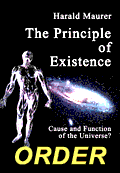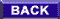The gravitational force which two bodies seem to exert upon each other manifests in an accelerated motion towards one another. Galileo was the first to discover that in the gravitational field of the Earth all bodies fall at the same speed. This had to be a mystery considering the different weights of the bodies. Galileo’s laws of falling bodies and Kepler’s laws made Newton establish that the cause of the equal acceleration of all bodies was to be found in an attraction between the masses which makes both the apple fall from its tree and keeps the moon in its orbit around the Earth. He realised that the force between the falling mass m1 and the mass of the Earth m2 had to be proportional to m1 (because of F=ma it follows F/m=a=const) and that an equally strong opposite force was acting on mass m2 and that therefore F had to be proportional to m2 as well. Consequently this means for the dependence of the gravitational force on the two masses involved: F is proportional to m1m2 .  In other words: F=ma expresses the inertia of the falling body, the mass results from inertia and exerts a force upon the mass of the Earth which is identical with the one that the mass of the Earth exerts on the falling body.If ever a conclusion has been purposeful in science it was this one. For surely the Earth can never know what mass is just falling down on it and adjust its force to the inertia that is coming down. This automatic adjustment of forces is strange because gravitation is a symmetrical phenomenon which always only occurs between at least 2 masses in which case the masses are the cause of gravitation (“gravitational mass“), whereas one mass alone represents the inertia (“inertial mass“) and these masses are obviously equivalent, i.e. have exactly the same properties. This means: one cannot find out if a force acting on a mass has been caused by the gravitational force or if it occurs as inertia in an accelerated frame of reference. This insight was therefore the starting point for a relativistic theory of gravitation: Einstein’s General Theory of Relativity.     Einstein put an end to gravitation by - tersely said - “curving” the space, equating the gravitational mass with the inertial mass (i.e. adopting the principle of equivalence from Newton)  and just letting it fall down the curved space – and in doing so, the concept of “mass“ was somehow lost along the way. We already underlined that Einstein discovered the “mirror image of reality“ with that (because masses and attraction forces do not really exist after all).  But before we deal with this mirror image (in the chapter about “Relativity“) we will deal with reality.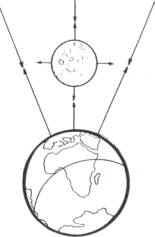Fig.81AFig.81a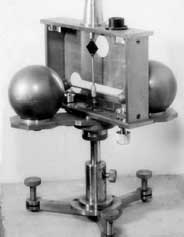81b     When we take a look at figure 81 we should ask ourselves what applies more pressure (or maybe we should rather say thrust) on the moon (which is not true to position and scale here) – the Earth or the universe? Since the whole (!) universe is all around the Earth and the moon there is only one answer: without any doubt the pressure from the universe is considerably stronger. Since the weaker pressure from the Earth is acting against it the moon is not in equilibrium with its environment. But it is possible to  immediately detect when the moon makes a movement in direction of the Earth, which the moon is certainly forced to do by the force of the universal pressure. Well, the universal pressure does not stop being an applied force, and therefore the moon receives an acceleration as defined by us in the chapter about “Inertia“. In this example, it is the  Earth’s acceleration of free fall; as everybody knows, it is 9.81 m/s2. (In the next chapter we will learn why the moon doesn’t just fall down.)     The field of the falling body is only in equilibrium with the forces acting all around it and therefore weightless when it has this acceleration. When we (or the Earth’s surface) disturb this equilibrium by preventing the compensation of movement, the force of the universal pressure results in a noticeable pressure between the  surface of the Earth (or a pair of scales!) and the field. This pressure can be measured, its quantity is called weight. At the bottom of this casual mode of expression lies that complicated dynamic effect which we discovered in the inertia: the transmission of the thrust force of the Earth’s field through the “falling” body at the velocity of light and the transmission of the thrust force of the universal pressure through  the same body at the velocity of light from the other side! One can assume that the nature of the body does not play a significant role for this transmission of power and that it only determines the density, the impression of the mass,  and the gravitational magnitude. We therefore require a factor for the ratio between the density (which can of course be just the same for a little stone as for a giant star!) and the gravitational magnitude which expresses this proportional ratio, i.e. a gravitational constant because without this constant the law of gravitation would be completely worthless!     And here is the next strange fact: that one was really looking for such a constant in view of the variety of masses in the universe and the variety of gravitational effects allegedly caused by them and - even stranger - really found one! Because according to Newton’s law it is not possible at all to discover a constant in the range of the Earth which is to apply throughout the universe! Because his law only describes the relation of two masses with a god-given force (because it is not logically comprehensible). For that reason, the constant would have to be given by God as well and to be inherent to matter in the same mysterious way as gravitation itself. Can one measure such a thing?      One could. And one could do it only because the universe is really filled with a measurable force. It is the universal pressure! Cavendish measured the force of the universal pressure when he established the constant of gravitation. It was not the force of attraction between the masses of his gravitational or torsion balance. Let’s go deeper into the matter (fig. 81a. Fig. 81b shows the original apparatus):      When the two bigger spheres are approached by the smaller spheres the latter are compressed by the universal pressure. The force applied to shove the smaller spheres leads to a twisting of the torsion wire until its resilience equals the thrust force. The torsion is shown on the graduation. Now the two bigger spheres are moved to the other side of the smaller spheres. In doing so the smaller spheres are shoved in the opposite direction. The constant of gravitation can now be calculated by means of the law of gravitation: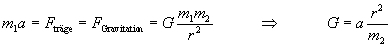There have been various modifications and improvements of the measuring methods. In principle, it is always the acceleration of two test masses which is measured in comparison to each other. This acceleration is nothing but the inertia being surmounted by the universal pressure, i.e. by means of the universal field which is composed of the tiniest shoves pulsating along at the velocity of light. And this field  acts upon a body which allows being affected, invaded, or penetrated at the velocity of light at the most. If one tries to measure a proportional action factor in this event between two “inert masses”, one will measure nothing else but  the relation of these velocities to each other! When we take the reciprocal value of c, i.e. 1/c, as quantity for the resistance against the force penetrating at c (= velocity of light), we obtain  the factor for the inertia for one mass. As a result, the factor for the gravitational proportionality of two masses is therefore 2 x 1/c = 2/c. (1/G would be the quantity for “rigidity” of space and time in the General Theory of Relativity). The gravitational force at the surface of a mass of 1 kg (density 1) is about 6.6713*10-9  N, i.e. twice the reciprocal value of velocity of light 6.6713*10-9 m/s. This numerically astonishing correspondence has already been noticed by some astronomers but they will probably take if for coincidence. It doesn’t seem to be coincidence, though. But one should not take the figures too seriously either because the constant G is not precisely known (and presumably it is not even a constant at all),  and although the constant c is known quite exactly – nobody has ever proved whether  it is a constant (there aren’t any “natural constants“ after all). The connection of c with gravitation, however, does not only become  even clearer in the General Theory of Relativity but it actually takes on a fundamental significance. And before we jump right into the theory with our train of thought we will consider the background of Newtonian dynamics in a more superficial manner.     Every falling body regulates the power of application from the Earth’s pressure  and the universal pressure by means of its size (the size of the field of resistance). That means the universal pressure affects a small body with less force, and the Earth exerts a lower counter-pressure, too. A big body is affect with more force by the universal pressure - and the counterforce of the Earth is also stronger! Because of this automatic compensation all bodies fall certainly at the same speed! And we have to accept that heavy mass action seems to be indeed the same as accelerated inert mass action – which is not surprising because the heavy mass action does not exist as the cause of gravitation at all. Thus there only exists some kind of “mass“ - and it is only an effect and not a “primordial matter“ of which matter could possibly consist. For that reason, Newton’s “quantity of matter“ strikes us as being so applicable because it could also be translated as “quantity of the event(s)”! And that is how it really is: we are not dealing with objects but with events.      The weight of a body reveals to what extent it looses the equilibrium with its environment. When the compensation of the counteracting Earth pressure is gone, the bodies previously falling at the same speed are suddenly of different weight, of course. Thus this gravity is defined immediately by the quantity of energy representing the field through its (lack of) motion. We call this quantity potential energy. It is absolutely identical with the kinetic energy and to be equated with it as we already discovered in our example of the fan wheel.     In fact, a moving body carries the exerted force that is moving it but it appears to be free from a force when the motion is uniform because the universal pressure acting against it compensates this force. When the motion and with it the compensation stops the force is released again and performs further  work. Since the internal content of energy - as we noticed when we discussed the inertia - was really increased by modifications to the impulse which also increased the inertia by the amount of this force - which in turn increased the action of mass - the physicist can simply calculate the energy carried along by means of the mass of a field and its velocity. However, we have to keep to half the mass (1/G!), i.e. kinE=l/2m*v2.     A body that hits the ground and burrows a big hole receives its energy for doing so directly from the cosmos. Once exerted, a force can be transmitted from body to body without loosing energy. This is known as the conservation of the impulse. And for that reason, we have called any disturbance of T.A.O. impulse from the beginning... And now the question is: Do really all bodies fall down to earth at the same speed without exception? The astonishing answer to this question is no! Because we must not fail to notice an important factor in the game of universal pressure and Earth pressure: the geometrical arrangement of the forces in relation to each other and the curving force resulting thereof! As we already know this force originates from the tendency of a body to resist deformation. Something very similar applies to the inertia, too, if we remember our discovery that every body is fundamentally deformed by the exerted forces!       In a space with a given curvature, like the space around the Earth, the magnitude of the curving force results from the relation of two fields to one another. Well, we realised that the acceleration of free fall stems from the relation between Earth pressure and universal pressure. Actually a constant acceleration should only arise if these two opponents were of the same size in every place and all the time. But they are not.     Our figure 15 already illustrated the geometry of the distribution of pressure. The farther we get away from Earth, the weaker the universal pressure becomes oddly enough. Its field lines are getting thinner as it were (the reason for this is that the pressure streams in spherically). The closer we get to the Earth, the stronger the Earth’s pressure becomes. The field is getting denser. Since the field of the falling body is situated between these forces, there are always a few more lines of force of the Earth pressure acting on the field than lines of force of the universal pressure. This would result in a constant slight surplus for the Earth pressure if the curving force did not intervene and compensate this difference. The curving force of a body falling to Earth, however, is conditional on its expansion. Generally speaking that is the reason why dense materials are actually always a little lighter than those which are loosely bonded (a fact which physicists have been unable to explain). And therefore bodies which are expanding are getting a little bit heavier! In a very big falling field the curving force becomes so strong that it will break the body before it falls down to Earth. The rings around the planets, of which we will speak later-on, are created in this manner. And a difference between vertical and horizontal masses is the result which has been unknown so far.     On the other hand, however, a very small field does not feel much of the curving force of the Earth. And now the Earth’s pressure wins the game: the very small field will not fall down to Earth - and if it came into existence on the Earth’s surface, the Earth would send it into the universe immediately!     Thus a field of this kind would have to be very small, and in addition it would not allow to be bound to other fields. There is only one such small, independent field on Earth: helium! And for the described reason helium is continuously disappearing from Earth without leaving a trace although it is produced in vast amounts by the radioactivity of the Earth’s rocks. For the same causes, helium flows upwards on the wall of every containing vessel without bothering about gravitation. This “mysterious” state is called superfluid - and we see that this property is not so mysterious at all. Hydrogen is catapulted into space as well when it occurs separately in the upper layers of the atmosphere. But luckily hydrogen loves to bond and so quite a bit is preserved. Well, we should think of the pressure and the composition of the solar wind and of all those vast amounts of particles which are sent on a journey into infinity by the stars of this universe because they are small enough to escape from the lurking curving forces of the cosmos...     We are not yet finished with the subject of gravitation by a long shot. Many phenomena which have been regarded as mysteries of physics up to now can be unravelled by turning gravitation upside down. For example, it is absolutely impossible to explain by means of gravitation why celestial bodies or actually all masses are gravitating leisurely for infinitely long and why they are exerting attraction forces onto each other without receiving any energy from anywhere! Even Einstein’s masses are quite occupied with the curvature of space - and nobody knows where they get the energy from in order to do so! These gravitational theories have a mystical component: no matter if they generate gravitation without supply and regeneration of energies or if they show other masses in Einstein's space-time which curved paths they have to fly on in order to age as strongly as possible (yes, we will not be spared to examine this one, too) by means of using energies of mysterious origin as well, basically they are just not logically comprehensible.      The repulsion principle does not allow for this dilemma in explanation. The displacement, the repulsion, or the pressure, they are achieved after all by the energy flux from one body to the other. We will come to see that this energy flux is the motor which actually keeps the whole universe going. * Newton himself had his problems with his thesis. He wrote: " That gravity should be innate, inherent and essential to matter, so that one body should act upon another at a distance, through a vacuum, without the mediation of anything else, by and through which their force may be conveyed from one to another, is to me so great an absurdity that I believe no man who has, in philosophical matters, a competent faculty of thinking, can ever fall into it."Menu Sitemap Start Download Order